# AP Board 9th Class Physical Science Solutions Chapter 8 Gravitation

AP State Syllabus AP Board 9th Class Physical Science Solutions Chapter 8 Gravitation Textbook Questions and Answers.

## AP State Syllabus 9th Class Physical Science Solutions 8th Lesson Gravitation

### 9th Class Physical Science 8th Lesson Gravitation Textbook Questions and Answers

Question 1.
What path will the moon take when the gravitational interaction between the moon and earth disappears? (AS 2)
The force of attraction between moon and earth is given by F = $$\frac{\mathrm{GMm}}{\mathrm{R}^{2}}$$
M = mass of the earth ; m = mass of the moon ; R = radius of the earth
Here the gravitational interaction between moon and earth disappears.
∴ G = 0 ⇒ F = 0

• Therefore the moon neither revolves around the earth nor fall into the earth.
• It takes a straight path away from the earth.

Question 2.
A Car moves with constant speed of 10 m/s in a circular path of radius 10 m. The mass of the car is 1000kg. Who or what is providing the required centripetal force for the car? How much is it? (AS 1)
Speed of the car (v) = 10 m/s ; Radius of the path (r) = 10 m
Weight of the car (m) = 1000kg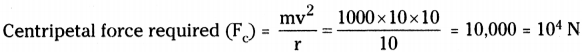The required centripetal force is provided by the friction between the tyres of the car and the road.

Question 3.
A small metal washer is placed on the top of a hemisphere of radius R. What minimum horizontal velocity should be imparted to the washer to detach it from the hemisphere at the initial point of motion? (AS 1, AS 7)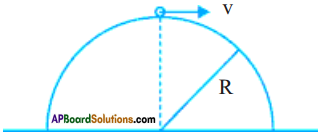Radius of hemisphere = R; Mass of hemisphere = M
Let the radius of washer = r and mass = m
Distance between hemisphere and washer = R + r
The centripetal force required to rotate the washer = $$\frac{\mathrm{mv}^{2}}{\mathrm{r}}$$
The gravitational force of washer due to hemisphere is = $$\frac{\mathrm{GMm}}{\mathrm{R}^{2}}$$
But the necessary centripetal force must be equal to the gravitational force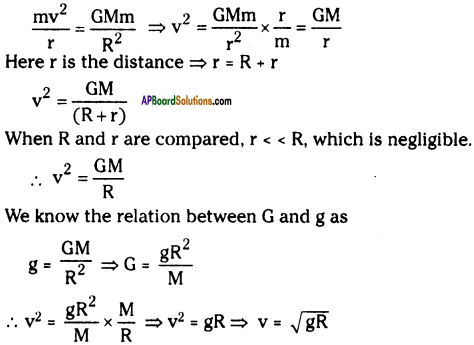Question 4.
Explain why a long pole is more beneficial to the tight rope walker if the pole has slight bending. (AS 1, AS 7)

• You must have noticed the circus artists doing rope walking.
• During this act, they carry a long bamboo pole in their hands.
• The reason for this is that the line joining the centre of gravity and centre of equilibrium must fall within the rope for achieving the stable equilibrium.
• Thus when an artist finds that he is falling towards left, he shifts bamboo pole towards right, so that his centre of gravity stay undisturbed.
• Thus he can balance himself on the rope.Question 5.
Why is it easier to carry the same amount of water in two buckets, one in each hand rather than in a single bucket? (AS 7)
It is because in the later case centre of gravity of our body shifts towards the bucket and there is a tendency that the line joining the centre of gravity and centre of equilibrium may fall outside our feet.

However in the former case the centre of gravity not only gets lowered, but also it is at such a point that line joining the C.G. and C.E. falls within our feet.
Hence one is a stable equilibrium.

Question 6.
What is the speed of an apple dropped from a tree after 1.5 second? What distance will it cover during this time? Take g = 10 m/s². (AS 1)
An apple is dropped from a tree.
∴ Initial velocity u = 0 ; Time t = 1.5 s
a = g = 10 m/s2 ; Final velocity v = ?
v = u + at = 0 + 10 x 1.5 = 0 + 15 = 15 m/s
Distance covered (s) = ut + $$\frac{1}{2}$$ at² = 0 × 1.5 + $$\frac{1}{2}$$ × 10 × 1.5 × 1.5 = 0 + 5 × 2.25 = 11.25 m

Question 7.
A body is projected with a speed of 40 m/s vertically up from the ground. What is the maximum height reached by the body? What is the entrie time of motion? What is the velocity at 5 seconds after the projection? Take g = 10 m/s². (AS 1)
Initial speed u = 40 m/s ; g = 10 m/s²
Maximum height reached (h) = $$\frac{\mathrm{u}^{2}}{2 \mathrm{~g}}=\frac{40 \times 40}{2 \times 10}$$ = 80 m
Entire time of motion (T) = $$\frac{2 \mathrm{u}}{\mathrm{g}}=\frac{2 \times 40}{10}$$ = 8 s
Entire time of motion is 8 seconds.
∴ It starts to fall down after 4 seconds.
At 5 seconds the body is in downward direction.
u = 0 m/s, a = g = 10 m/s², t = 5 – 4 = 1 sec.
v = u + at = 0 + 10 × 1 = 10 m/s
∴ The velocity at 5 seconds is 10 m/s downward.Question 8.
A boy is throwing balls into the air one by one in such a way that when the first ball thrown reaches maximum height he starts to throw the second ball. He repeats this activity. To what height do the balls rise if he throws twice in a second? (AS 1, AS 7)
The boy throws the second ball when the first ball reaches its maximum height. He throws twice in a second.
Time of ascent of first ball is 1/2 sec.
After 1/2 sec, the first ball starts to fall down and the second ball starts from ground.
Let the distance travelled = s meters.; Initial velocity = u m/s
Time of ascent (t1) = 1/2 sec.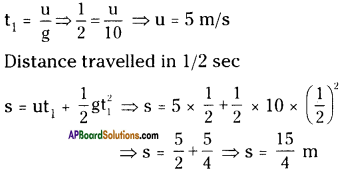They reach a height of 15/4 meters.

Question 9.
A man is standing against a wall such that his right shoulder and right leg are in contact with the surface of the wall along his height. Can he raise his left leg at this position without moving his body away from the wall? Why? Explain. (AS 7)

• When the right leg and right shoulder are in contact with the surface of the wall along the height of a man, his weight is towards the wall.
• The centre of gravity will be away from the foot. Just like in the case of carrying a bucket full of water with one hand.
• The line joins the centre of gravity and centre of equilibrium is not perpendicular to the horizontal.
• Hence he cannot lift his left leg without moving his body along the wall.

Question 10.
A ball is dropped from a height. If it takes 0.2 s to cross the last 6 m before hitting the ground, find the height from which it is dropped. Take g = 10 m/ s². (AS 1)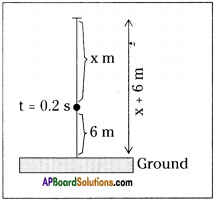For last 6 m, distance travelled
s = 6 m ; u = ? ; t = 0.2 sec ; a = g = 10 m/s²
s = ut + $$\frac{1}{2}$$ at²
6 = u (0.2) + $$\frac{1}{2}$$ × 10 × (0.2)²
6 = (0.2) u + (5 × 0.04)
6 – 0.2 = 0.2 u
5.8 = 0.2 u ⇒ u = $$\frac{5.8}{0.2}$$ = 29
∴ u = 29 m/s
This u will be the final velocity while travelling the distance x.
∴ s = x, v = 29 m/s, a = g = 10 m/s², u = 0 m/s
v² – u² = 2as ⇒ 29² – 0 = 2 × 10 × x ⇒ x = $$\frac{841}{20}$$ = 42.05 m
∴ Total distance = x + 6 = 42.05 + 6 = 48.05 m
∴ The ball is dropped from a height of 48.05 m.

Question 11.
A ball is dropped from a balloon going up at a speed of 5 m/s. If the balloon was at a height 60 m. At the time of dropping the ball, how long will the ball take to reach the ground? (AS 1, AS 7)
At t = 0, the stone was going up with a velocity of 5 m/s. After that it moves as a freely falling body, with downward acceleration ‘g’.
If it reaches the ground at time t1,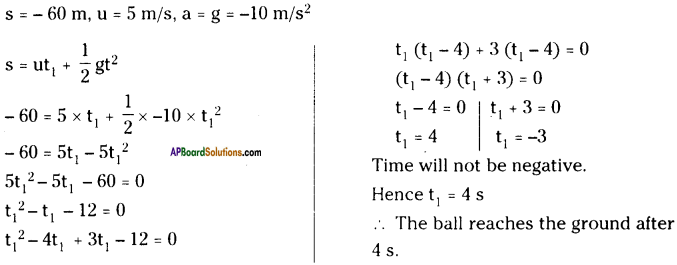Question 12.
A ball is projected vertically up with a speed of 50 m/s. Find the maximum height, the time to reach the maximum height, and the speed at of the maximum height. (g = 10 m/ s²) (AS 1)
Initial speed u = 50 m/s ; g = 10 m/s²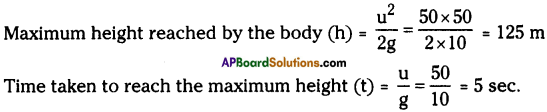After reaching maximum height, the velocity becomes ‘zero’.

Question 13.
Two cars having masses m1 and m2 move in circles of radii r1 and r2 respectively. If they complete the circle in equal time. What is the ratio of their speeds and centripetal accelerations? (AS 1)
Masses of cars : m1 and m2; Radius of circles : r1 and r2
Given that their time period is equal.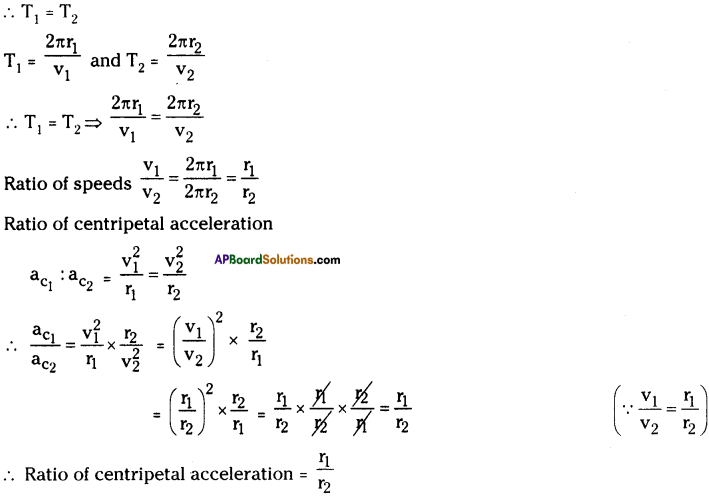Question 14.
Two spherical balls of mass 10 kg each are placed with their centers 10 cm apart. Find the gravitational force of attraction between them. (AS 1)
Masses of balls M1 and M2 = 10 kg each.; Distance d = 10 cm = 0.1 m
Gravitational force of attraction between them is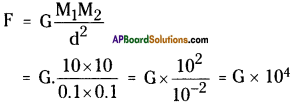Question 15.
Find the free-fall acceleration of an object on the surface of the moon, if the radius of the moon and its mass are 1740 km and 7.4 × 1022 kg respectively. Compare this value with free fall acceleration of a body on the surface of the earth. (AS 1)
Radius of the moon = 1740 km = 1740 × 10³ m
Mass of the moon = 7.4 × 1022 kg ; G = 6.67 × 10-11 Nm² kg-2
Free fall acceleration of a body on the surface of the moonQuestion 16.
Can you think of two particles which do not exert gravitational force on each other? (AS 2)
Two particles which do not exert gravitational force on each other will be mass less particles. But every particle has even a little mass. Hence we cannot find two particles which do not exert gravitational force on each other.

Question 17.
An apple falls from a tree. An insect in the apple finds that the earth is falling towards it with an acceleration g. Who exerts the force needed to accelerate the earth with this acceleration? (AS 7)

• According to Newton’s third law, when an apple is freely falling, the force on the apple due to earth is equal to the force on the earth due to apple.
• The force is due to gravity, which causes acceleration in the body.
• As the insect is inside the freely falling apple, it feels that the earth is falling towards it with an acceleration ’g’.
• According to the insect, the acceleration is due to the force on the apple due to the earth.
• Actually the earth is not falling towards the apple.Question 18.
A scooter weighing 150 kg together with its rider moving at 36 kin/hr is to take a turn of radius 30 in. What force on the scooter towards the center is needed to make the turn possible ? Who or what provides this? (AS 1)
Weight of the scooter with rider = 150 kg
Speed = 36 km/hr = 36 × $$\frac{5}{18}$$ = 10 m/sec.
Radius of the turning = 30 m
The force needed on the scooter towards the centre is centripetal force.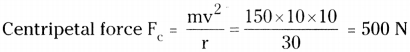∴ A centripetal force of 500 N is required on the car.
This force is provided by the friction between the tyres of the car and road.

Question 19.
The bob of simple pendulum of length 1 m has mass 100 g and a speed of 1.4 m/s at the lowest point in its path. Find the tension in the string at this moment. (Take g = 9.8 m/sec²) (AS 1)
Mass of the bob = 100 g = 0.1 kg ; Length of the string = 1 m
Speed of the bob v = 1.4 m/s ; Let the tension in the string be T.
The forces acting on the bob are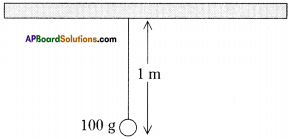a) Weight of the bob mg, downwards
b) Tension in the string ‘T’ upward
Weight of bob = $$\frac{\mathrm{mv}^{2}}{\mathrm{l}}$$
Tension in the string T = g cos θ
∴ According to Newton’s third law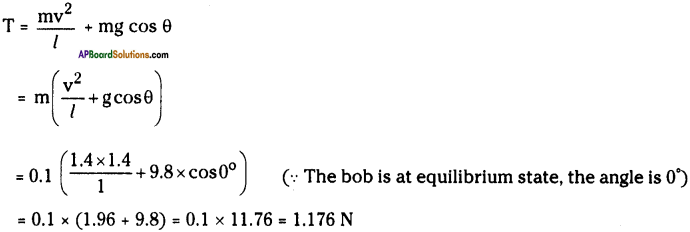Question 20.
How can you find the centre of gravity of India map made with steel? Explain. (AS 3)
(OR)
Describe an activity to know the center of gravity of a India map.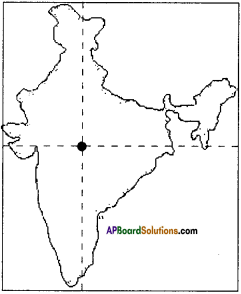1. Take an India map made of steel.
2. Make holes at four different corners i.e., top, bottom, left, and right, and name them as A, B, C, and D as shown in figure.
3. Suspend the map through a hole A, by means of a string to a nail ‘P’ as shown in figure.
4. Suspend a plumb line from the nail P.
5. Draw the line AX along the plumb line.
6. Similarly suspend the map through other holes B, C and D and draw lines BY, CZ, DW along the plumb line.
7. These lines (more than two) intersect at a point.
8. This point (G) is the centre of gravity of the map.

Question 21.
Explain some situations where the center of gravity of man lies out side of the body. (AS 1)
Centre of gravity of a human being is located interior to the second sacral vertebra.
Centre of gravity of man lies out side the body in the following situation :
a) While doing sit-ups.
b) While carrying a load like bucket full of water with one hand.
c) While walking on a narrow base like walking on a rope or pole or narrow wall, etc.
d) While walking with one leg.
e) As age increases its position changes.Question 22.
Where does the centre of gravity of atmosphere of the earth lie? (AS 2)
The earth’s atmosphere is about 10,000 km thick, but most of its bulk is contained in the first 11 km above the earth’s surface. Since the earth and its atmosphere are roughly spherical, the centre of the earth is also the centre of gravity of the earth’s atmosphere.

Question 23.
Where does the center of gravity lie, when a boy is doing sit-ups? Explain. (AS 7)

• The centre of gravity of a boy when he start erect, falls in the foot.
• When he is doing sit-ups, the centre of gravity shifts from foot to his base.
• The weight vector also move from the base.
• Hence the boy stretches his hands or bends slightly towards the earth. While doing sit-ups, in order to make the weight vector pass through base, so that he acquire stability.

### 9th Class Physical Science 8th Lesson Gravitation InText Questions and Answers

9th Class Physical Science Textbook Page No. 126

Question 1.
What is that force?
The force acting on these objects to make them move around another object, instead of moving in straight line in the gravitational force.

Question 2.
Is the motion of the earth around the sun uniform motion?
The earth takes 365.25 days to complete one rotation around the sun. Hence it is uniform motion.Question 3.
Is the motion of the moon around the earth uniform motion?
Moon takes 27.3 days to complete one rotation around the earth, which does not change. Hence it is uniform motion.

9th Class Physical Science Textbook Page No. 127

Question 4.
Does the velocity of the body change in uniform circular motion? Why?
The velocity of the body in uniform circular motion is constant.

If the velocity is not constant, the time period changes from time to time and it cannot be treated as uniform circular motion.

Question 5.
Does the body in uniform circular motion have an acceleration? What is the direction of acceleration?
The body in uniform circular motion have an acceleration, which is directed towards the centre of the circle, known as centripetal acceleration.

9th Class Physical Science Textbook Page No. 129

Question 6.
Do you know what questions arose in his mind from this observation?
When apple fell to the ground, Newton might have thought like this:
a) Why did the apple fall on the earth?
b) Why doesn’t the apple go up in the sky?
c) Whether all the objects fall to ground ?
d) What makes them to fall on the ground?Question 7.
Why did the apple fall to the ground?
The apple fell to the ground due to the gravitational attraction of the earth.

Question 8.
Why does the moon not fall to the ground?
The gravitational force in the moon due to earth is equal to the gravitational force on the earth due to moon. Hence the moon does not fall to the ground.

Question 9.
What makes the moon to move in a circular orbit around the earth?
The gravitational force between moon and earth act as centripetal force and makes the moon to revolve around the earth in uniform circular motion.

9th Class Physical Science Textbook Page No. 135

Question 10.
How do you feel during the free-fall of your body from the height?

• I feel weightlessness during the free-fall of my body from the height.
• This is due to the gravitational force of earth on the body.

9th Class Physical Science Textbook Page No. 136

Question 11.
When the centre of gravity of the ladder and the centre of gravity of our body lies in the same line then we can balance the ladder on our shoulders.

9th Class Physical Science Textbook Page No. 128

Question 12.
Can an object moVe along a curved path if no force acts on it?

• If a body has to move in a curved path, its direction of velocity must change continuously.
• This is done by the centripetal force.
• Hence an object cannot move along a curved path if no force acts on it.Question 13.
As a car speeds up when rounding a curve, does its centripetal acceleration increase? Use an equation to defend your answer.
Centripetal acceleration $$a_{c}=\frac{v^{2}}{r}$$
As v increases, its centripetal acceleration also increases.

Question 14.
Calculate the tension in a string that whirls a 2 kg – toy in a horizontal circle of radius 2.5 iv when it moves at 3 m/s.
Mass of the toy m = 2 kg ; Radius of the circle = 2.5 m ; Speed of the toy = 3 m/s
As the toy is moving in a horizontal circle, the necessary centripetal force is provided due to the tension in the string.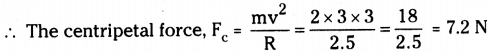9th Class Physical Science Textbook Page No. 131

Question 15.
In figure, we see that the moon falls around earth rather than straight into it. If the magnitude of velocity were zero, how would it move?If the magnitude of velocity were zero, the moon would move towards earth due to acceleration due to gravity.

Question 16.
According to the equation for gravitational force, what happens to the force between two bodies if the mass of one of the bodies doubled?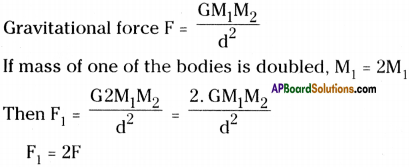∴ The gravitational force will be doubled.

Question 17.
If there is an attractive force between all objects, why we do not feel ourselves gravitating toward massive buildings in our vicinity?

• Earth is massive than the building.
• Hence the gravitational force between ourselves and earth is more than that of between ourselves and building.
• Hence we do not feel gravitating towards massive building in our vicinity.

Question 187.
Is the force of gravity stronger on a piece of iron than on a piece of wood if both have the same mass?
Yes. The force of gravity is stronger on a piece of iron then on a piece of wood if both have the same mass.Question 19.
An apple falls because of the gravitational attraction of the earth. What is the gravitational attraction of apple on the earth?

• We know that acceleration due to gravity, g = $$\frac{\mathrm{GM}}{\mathrm{R}^{2}}$$
Here M is the mass of the earth.
• In this mass of the object which is freely falling has no effect on ‘g’.
• Hence the gravitational attraction of apple on the earth is negligible.

9th Class Physical Science Textbook Page No. 133

Question 20.
Give an example for the motion of an object of zero speed and with non-zero acceleration?
Protons and neutrons inside the nucleus.

Question 21.
Two stones are thrown into air with speeds 20 m/s, 40 m/s respectively. What accelerations are possessed by the objects?
Stone -1 :
Initial velocity u = 20 m/s
After a time t, it reaches to ground then final velocity v = 0 m/s
Accelerahon = $$\frac{0-20}{t}=\frac{-20}{t} \mathrm{~m} / \mathrm{s}^{2}$$

Stone – 2 :
Initial velocity u = 40 m/s
Final velocity v = 0 m/s
Accelerahon = $$\frac{0-40}{t}=\frac{-40}{t} \mathrm{~m} / \mathrm{s}^{2}$$

9th Class Physical Science Textbook Page No. 135

Question 22.
When is your weight equal to mg?
When we are on the surface of the earth, our weight is equal to ‘mg’ on earth.Question 23.
Give example of when your weight is zero?

1. When we are at the centre of the earth, our weight is zero.
2. When we are freely falling, we feel weightlessness.

9th Class Physical Science Textbook Page No. 138

Question 24.
Where does the centre of gravity of a sphere and triangular lamina lie?
1) Centre of gravity of a sphere is a point where the whole mass of the sphere is assumed to be concentrated which is called centre.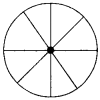2) Centre of gravity of a triangular lamina lies where the whole mass of the triangle is assumed to be concentrated which is centroid.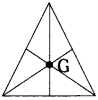Question 25.
Can an object have more than one centre of gravity?
Basing on the position or distribution of mass, an object can have more than one centre of gravity.

Question 26.
Why doesn’t the leaning tower of Pisa topple over?

• The centre of gravity of the leaning tower of Pisa is very close to earth.
• Even though it is leaning, the line of action of total weight passes through the base. The base area of leaning tower of Pisa is very large. So it doesn’t topple over.Question 27.
Why must you bend forward when carrying a heavy load on your back?
If we bend forward, then the line of action of total weight passes through the body. So we are stable. Hence we must bend forward when carrying a heavy load on our back.

9th Class Physical Science 8th Lesson Gravitation Activities

Activity – 1 Uniform circular motion

Question 1.
Describe an activity to observe uniform circular motion.
Uniform Circular Motion :
Uniform circular motion is a motion of the body with a constant speed in circular path.

Material required :
Electric motor, old C.D., small wooden block, battery, connecting chords, stop clock.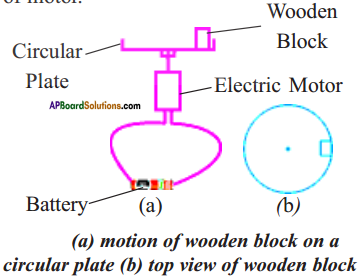Procedure:

1. Take an electric motor and fix a disc to the shaft of the electric motor.
2. Place a small wooden block on the disc, as shown in figure.
3. Switch on the motor.
4. Find the time required to complete ten revolutions by the block.
5. Repeat the same two to three times.
6. We observe that the wooden block moves in a circular path with a constant speed.
7. So, this motion of wooden block is called uniform circular motion.

Activity – 2

Question 2.
Define centripetal acceleration and derive an expression for centripetal acceleration.
Centripetal acceleration :
The acceleration which can change only the direction of velocity of a body is called centripetal acceleration.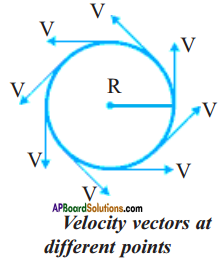Derivation :

1. Let a body is in uniform circular motion.
2. Draw velocity vectors at successive time intervals as shown in the figure.
3. Transfer tails of each velocity vector to coincide at a single point without changing their direction as shown in the figure.
4. In the above figure, the directed line joining two vectors represents change in velocity (Av).
5. Let us consider the change in velocity during the course of a complete revolution of a body i.e., the sum of the magnitudes of the changes in velocity during a complete revolution will be equal to the sum of the sides of the depicted polygon.
6. The smaller the sides of our polygon, the closer they cling to the circle of radius v, consequently the magnitude of change in velocity of the body during the course of revolution, will be equal to the circumference ’2πv’ of the circle.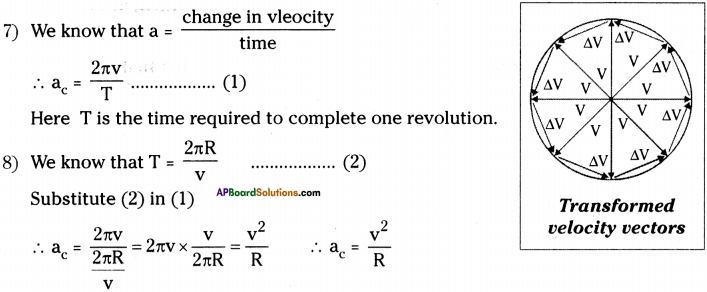9) As the vertex angle of isosceles triangle decreases, the angle between the change in velocity approaches to 90°.

10) Therefore, the acceleration of a body in uniform circular motion is directed perpendicular to its velocity.

Activity – 3 Free fall

Question 3.
Accelerahon is independent of masses.
Place a small paper on a book. Release the book with the paper from certain height from the ground.
The book and paper will fall on the ground at same time, if the paper is kept in the book. If the paper is kept on the book and released, then the paper will be separated from book and falls on the ground little bit later than the book.

b) Drop the book and paper separately, what happens?
Book reaches the ground first, then the paper reaches.Activity – 4

Question 4.
What is the direction of ‘g’?
Throw a stone vertically up. Measure the time required for it to come back to earth’s surface with stop clock.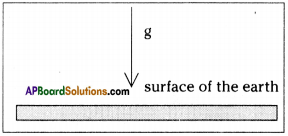a) What happens to its speed when it moves up and down?

• When the stone moves up, its velocity decreases gradually and finally it becomes zero, because it is moving against the direction of gravitation.
• When its velocity becomes zero, it starts to fall down due to gravitational force of earth, and its velocity increases.

b) What is the direction of acceleration?

• While the stone is moving up, the direction of acceleration is in upward direction, which is against to the direction of gravitational force.
• While the stone is falling down, the direction of acceleration is in downward direction, which is in the direction of gravitational force.

Activity – 5

Question 5.
Describe the method of measuring the weight of a free fail body.• Take a spring balance and suspend it to the ceiling and put some weight to it.
• Note the reading of the spring balance.
• Now drop the spring balance with load from certain height to fall freely.
• Carefully observe the change in the position of indicator on the spring balance scale while it is in free-fall.
• We observe that the indicator of spring balance shows zero while it is in free-fall.
• This is due to the weightlessness of a free-fall body.

Activity – 6

Question 6.
How do you demonstrate the changes during the free-fall of a body?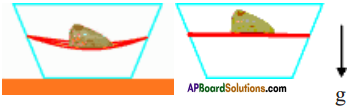• Take a transparent tray and make holes on opposite sides.
• Take two or three rubber bands and tie them tightly, close to each other between the holes.
• Now place a stone on the bands as shown in the figure.
• We observe that the bands bend due to the weight of the stone.
• Now drop the tray with stone.
• We observe that the bands do not bend. They straight.
• When in equilibrium on a firm surface, weight is evidenced by a support force. Then weight equals to mg.
• When the body falls freely then it experiences weightlessness.
• Even in this weightless condition, there is still a gravitational force acting on the body causing downward acceleration.Activity – 7

Question 7.
Give one activity that showing center of gravity and balancing.
(OR)
How do you balance spoon and fork?• As shown in the figure arrange a fork, spoon and wooden match stick together.
• The combination will balance nicely on the edge of the glass.
The weights of the spoon and fork will pass through the centre of gravity and the base (glass).

Activity – 8

Question 8.
Can you get up without bending?
Sit in a chair comfortably as shown in figure. Try to get up from the chair without bending your body or legs.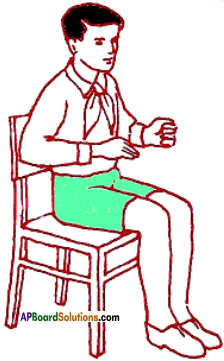a) Are we able to do so? If not why?

1. We cannot get up from the chair without bending our body or legs.
2. When we sit in a chair, we are in weightless condition.
3. We need a support force to get up from the chair.
4. This support force can be obtained by bending our body or legs.

Activity – 9

Question 9.

• Find the mid stick by counting the sticks of the ladder.
• Put that mid stick on the shoulder and balance the ladder.
• That is the center of gravity of a ladder.

Activity – 10

Question 10.
How can you locate the centre of gravity of a uniform object?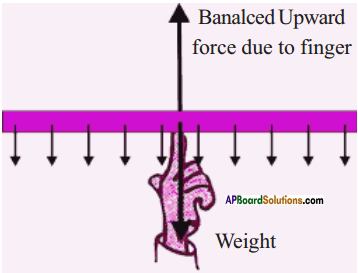1. Take a meter scale.
2. Suspend it from various points.
3. We observe that it bends to one side.
4. Suspend it from the mid point.
5. We observe that the scale will be in horizontal position without bending to any side.
6. At this point, the scale behaves as if its entire weight is concentrated at this point.
7. The support given at this single point gives support to the entire scale.
8. This point is its centre of gravity.

Activity – 11

Question 11.
Identifying the centre of gravity of a ring.
The center of gravity of any freely suspended object lies directly beneath the point of suspension.

If a vertical line is drawn through the point of suspension, the center of gravity lies somewhere along that line. To determine exactiy where it lies along the line, we have only to suspend the object from the some other point and draw a second vertical line through that point of suspension. The center of gravity lies where the two lines intersect.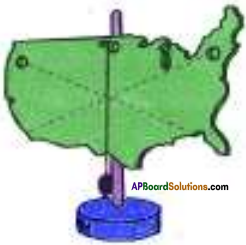a) Where does the center of gravity of a ring lie?
The centre of gravity of a ring lies at its geometric centre.

b) Does the center of gravity of a body exist outside the body?
For the bodies which are in right angled triangle shape, the centre of gravity falls out side the body. Hence we can say the CG of a body may be outside or inside the body.

c) Does center of gravity of an object exist at a point where there is no mass of the object?
The centre of gravity exist at a point where there is no mass of the object, for the objects like annual rings.Activity – 12

Question 12.
Shift of the centre of gravity and its effects.
Try to touch your toes as shown in figure (a). Try this again when standing against a wall as shown in figure (b).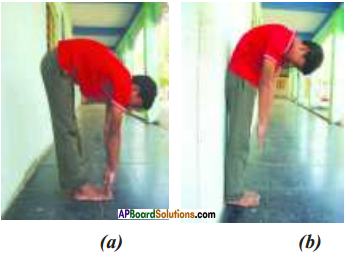a) Are you able to touch your toes in second case as shown in figure? If not why?